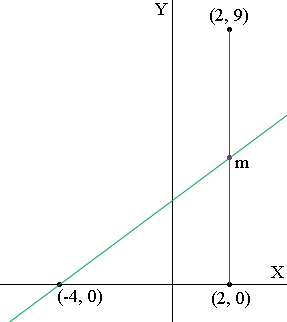SEARCH HOMEMath Central Quandaries & QueriesQuestion from Sara, a student: Line k lies in the xy-plane. The x-intercept of line k is -4. And line k passes through the midpoint of the line segment whose endpoints are (2,9) and (2,0). What is the slope of line k? Give the answer as a fraction.Hi Sara,

Did you draw a diagram?What are the coordinates of $m,$ the midpoint of the line segment from $(2, 0)$ to $(9, 0)?$

What is the slope of the line which passes through $(-4, 0)$ and $m?$

PennyMath Central is supported by the University of Regina and The Pacific Institute for the Mathematical Sciences.